If the following input signal is sent through a PN-junction diode, then the output signal across ${\mathrm{R}}_{\mathrm{L}}$ will be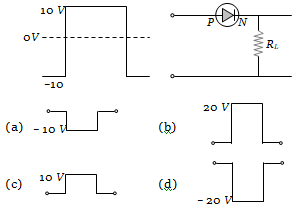Concept Questions :-

PN junction
High Yielding Test Series + Question Bank - NEET 2020

Difficulty Level:

The curve between charge density and distance near P-N junction will be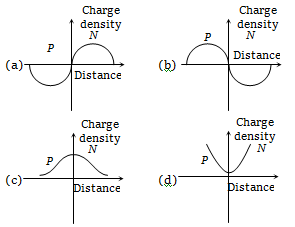Concept Questions :-

PN junction
High Yielding Test Series + Question Bank - NEET 2020

Difficulty Level:

The resistance of a germanium junction diode whose is shown in figure is $\left({\mathrm{V}}_{\mathrm{k}}=0.3\mathrm{V}\right)$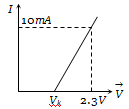(a) 5 k$\mathrm{\Omega }$
(b) 0.2 k$\mathrm{\Omega }$
(c) 2.3 k$\mathrm{\Omega }$
(d) $\left(\frac{10}{2.3}\right)\mathrm{k\Omega }$

Concept Questions :-

PN junction
High Yielding Test Series + Question Bank - NEET 2020

Difficulty Level:

In the half-wave rectifier circuit shown. Which one of the following wave forms is true for ${\mathrm{V}}_{\mathrm{CD}}$, the output across C and D?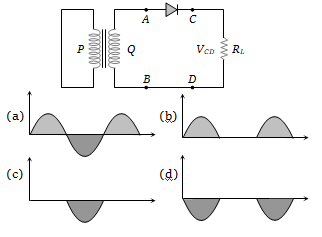Concept Questions :-

Rectifier
High Yielding Test Series + Question Bank - NEET 2020

Difficulty Level:

A full-wave rectifier circuit along with the input and output voltages is shown in the figure.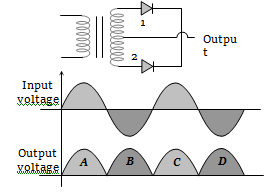The contribution to the output voltage from diode – 2 is :

(a) A, C                       (b) B, D
(c) B, C                       (d) A, D

Concept Questions :-

Rectifier
High Yielding Test Series + Question Bank - NEET 2020

Difficulty Level:

The i-V characteristic of a P-N junction diode is shown below. The approximate dynamic resistance of the P-N junction when a forward bias of 2 volt is applied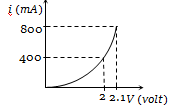(a) 1 $\mathrm{\Omega }$
(b) 0.25 $\mathrm{\Omega }$
(c) 0.5 $\mathrm{\Omega }$
(d) 5 $\mathrm{\Omega }$

Concept Questions :-

PN junction
High Yielding Test Series + Question Bank - NEET 2020

Difficulty Level:

The given figure shows the wave forms for two inputs A and B and that for the output Y of a logic circuit. The logic circuit is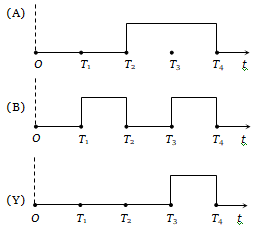(a) An AND gate                   (b) An OR gate
(c) A NAND gate                   (d) An NOT gate

Concept Questions :-

Logic gates
High Yielding Test Series + Question Bank - NEET 2020

Difficulty Level:

The variation of anode current in a triode corresponding to a change in grid potential at three different values of the plate potential is shown in the diagram. The mutual conductance of the triode is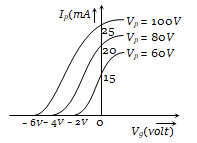(a) 2.5 m mho      (b) 5.0 m mho
(c) 7.5 m mho      (d) 10.0 m mho

Concept Questions :-

Transistor
High Yielding Test Series + Question Bank - NEET 2020

Difficulty Level:

A silicon speciman is made into a P-type semi-conductor by dopping, on an average, one Indium atom per $5×{10}^{7}$ silicon atoms. If the number density of atoms in the silicon specimen is  then the number of acceptor atoms in silicon per cubic centimetre will be

(a)                    (b)
(c)                    (d)

Concept Questions :-

Types of semiconductors
High Yielding Test Series + Question Bank - NEET 2020

Difficulty Level:

The probability of electrons to be found in the conduction band of an intrinsic semiconductor at a finite temperature

(a) Decreases exponentially with increasing band gap
(b) Increases exponentially with increasing band gap
(c) Decreases with increasing temperature
(d) Is independent of the temperature and the band gap

Concept Questions :-

Energy band theory